# How to make a simple water tank level indicator | Step by step instructions

## How to make a simple water tank level indicatorHello guys, welcome back. Today we will learn How to make a simple water tank level indicator. Also, this project mainly used the Arduino Uno board and the Ultrasonic sensor. If we talk a little bit about this project it can be used primarily for our home water tank. Also, we can monitor the water level using the LEDs in this project. We can also make this project at a minimal cost. This project can be made more effective by using a waterproof ultrasonic sensor instead of the ultrasonic sensor used for this project. But you can also use this HC-SR04 sensor. Also, the process of calculating the distance to an object by means of an ultrasonic sensor was used for this project.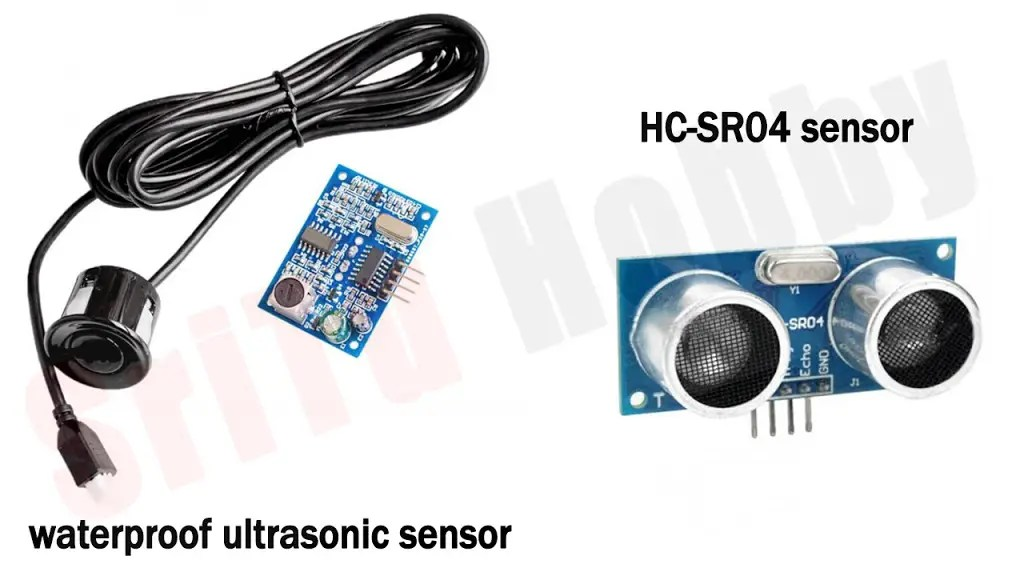OK, let’s do this project step by step. So, the required components are given below.

1. Waterproof sensor — Amazon
2. HC-SR04 sensor — Amazon / Banggood

### Step 1

Firstly, identify these components.

#### Arduino UNO board.#### Ultrasonic sensor.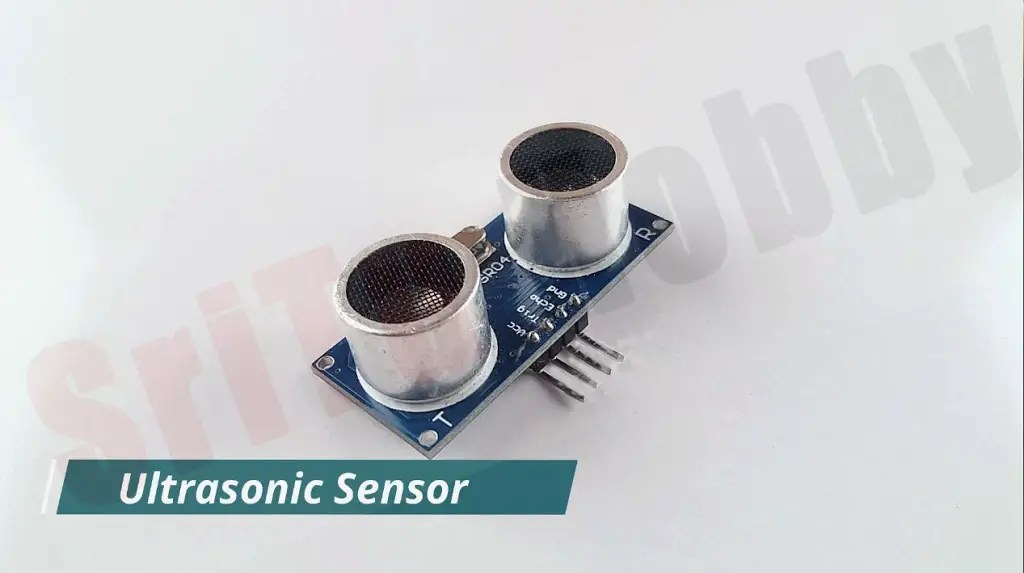#### Cardboard.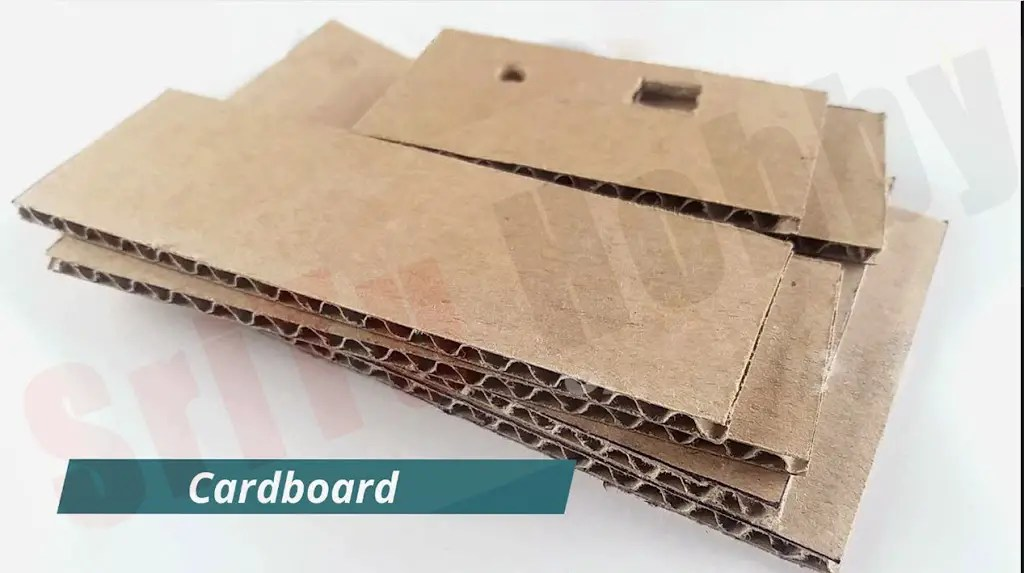### Step 2

Secondly, cut a 3 * 5-inch piece and a 3 * 1.5-inch piece. Then, glue these pieces together as follows. Use a glue gun for that.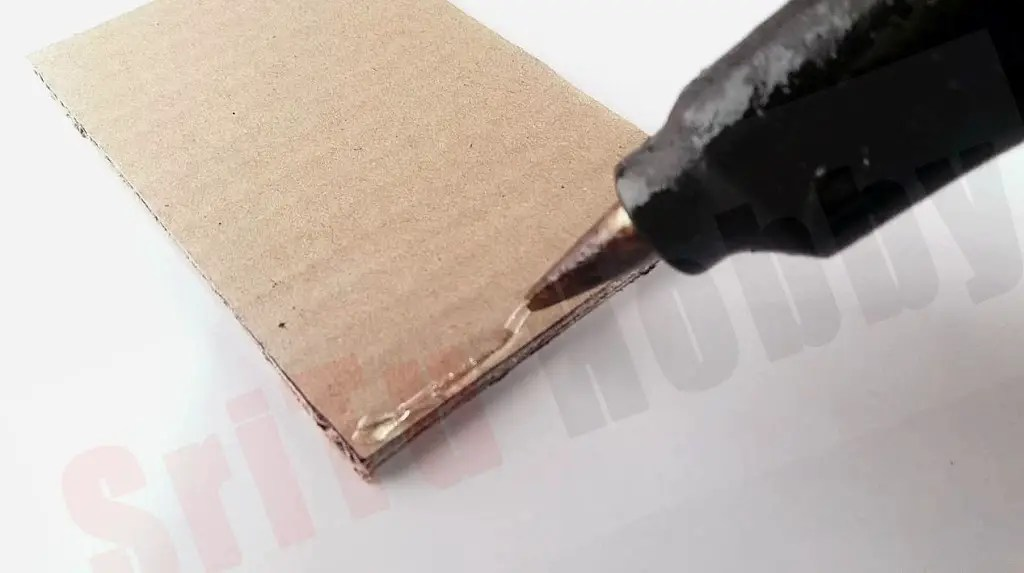### Step 3

Thirdly, attach the Arduino board to the bottom piece of cardboard.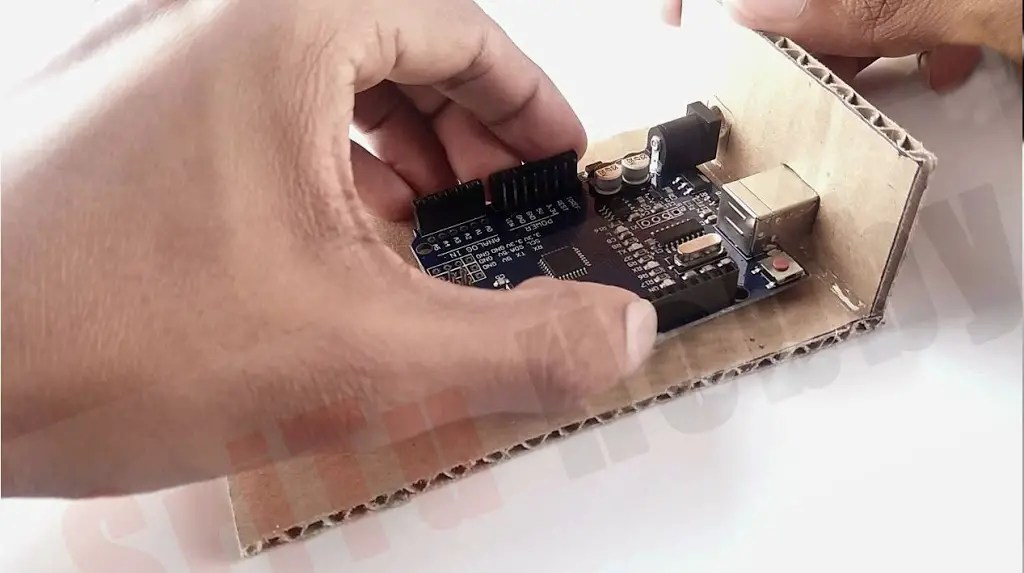### Step 4

Next, cut a 3 * 5-inch piece and attach the LED to it.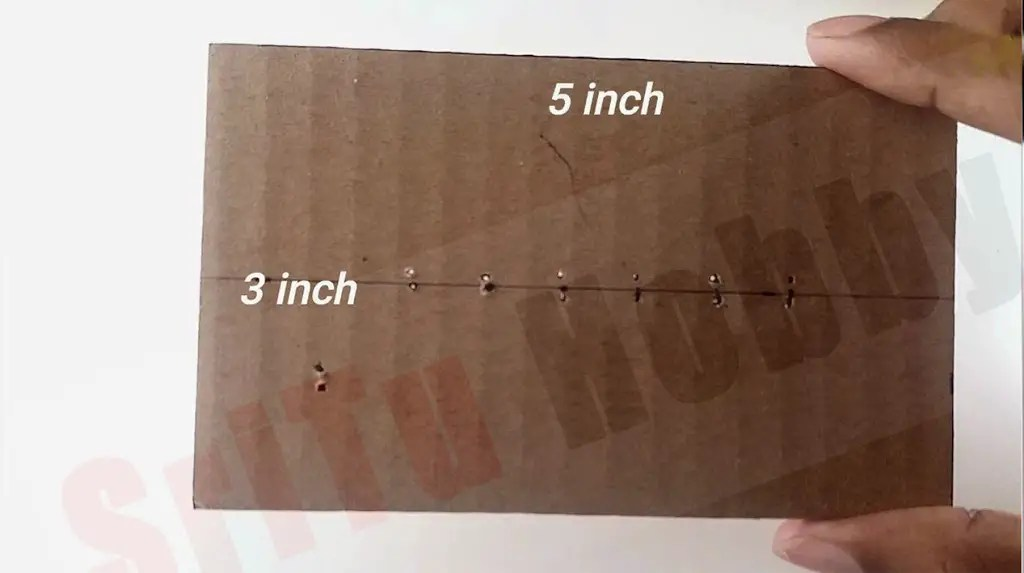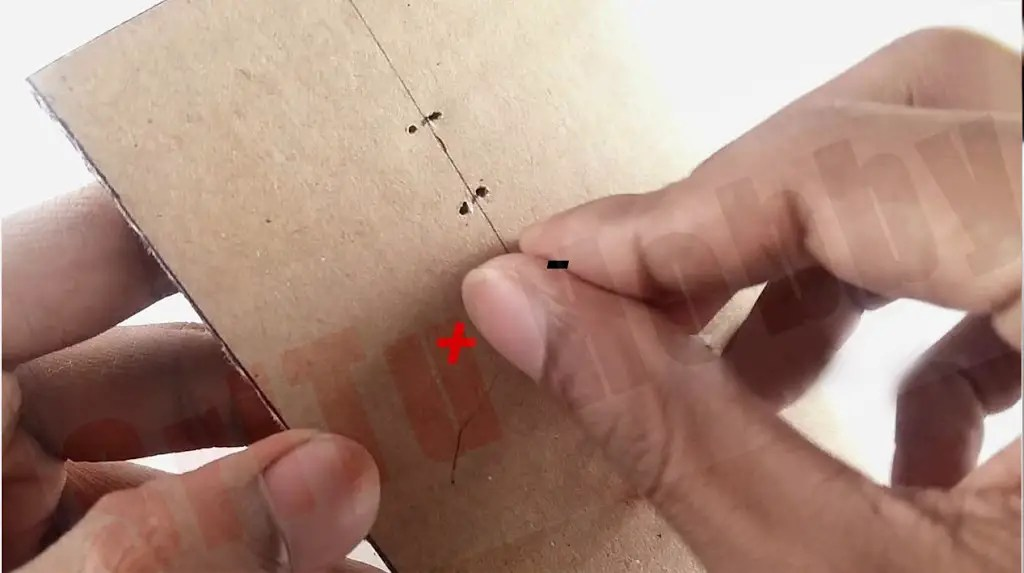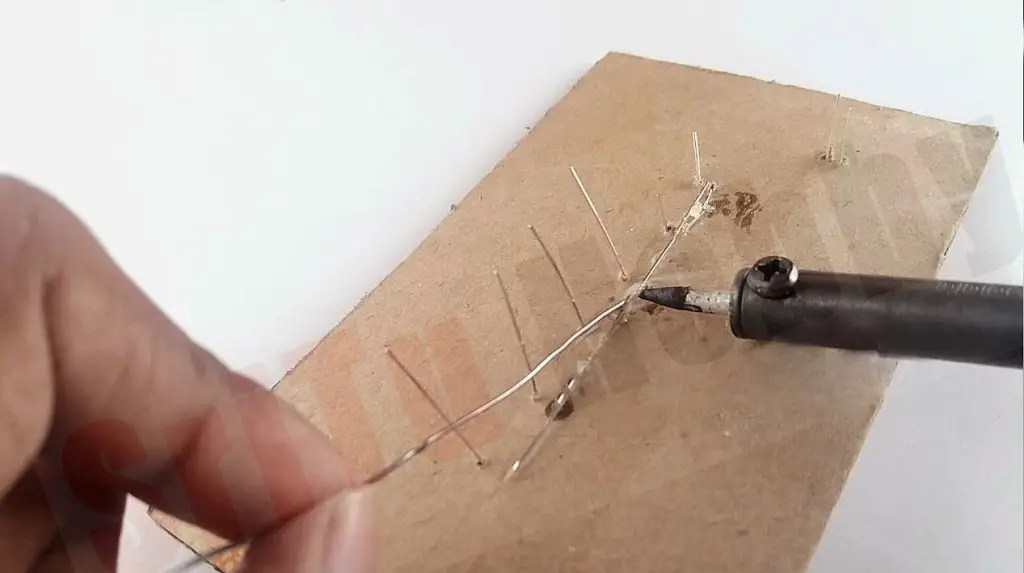### Step 5

Afterward, connect these LEDs and an Ultrasonic sensor to the Arduino board. For that use the circuit diagram below.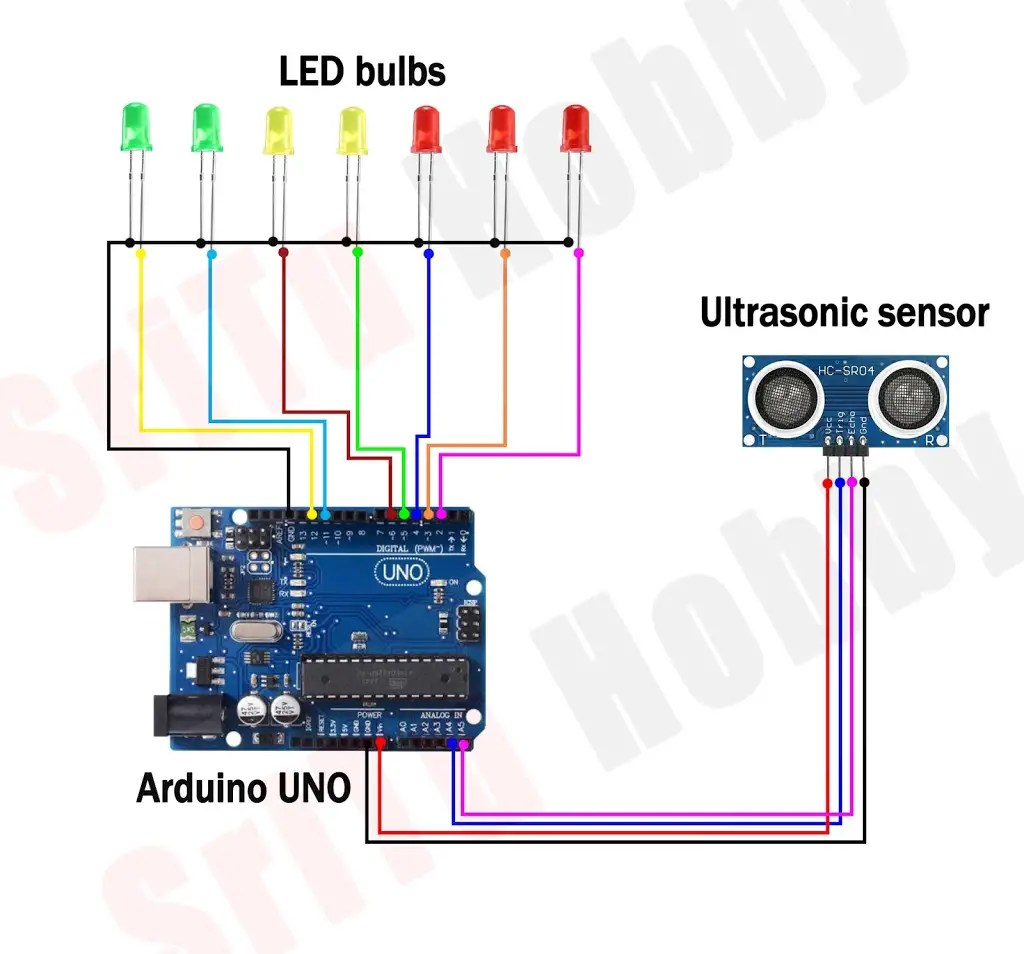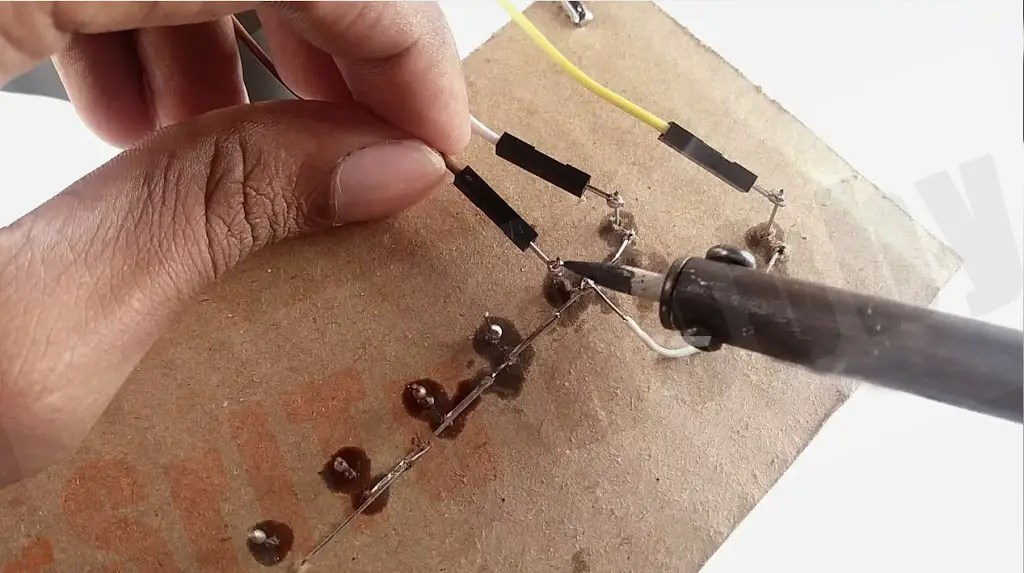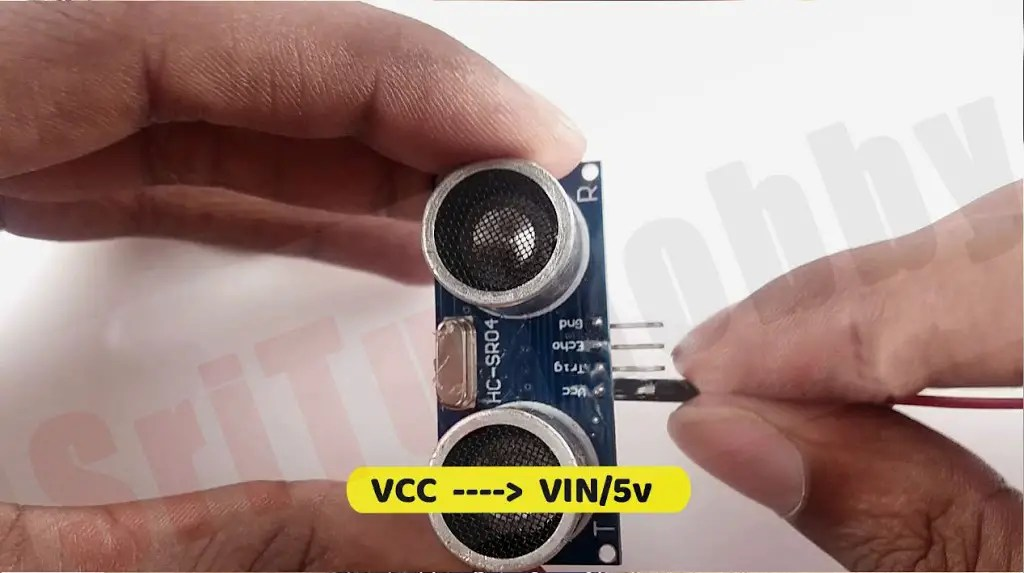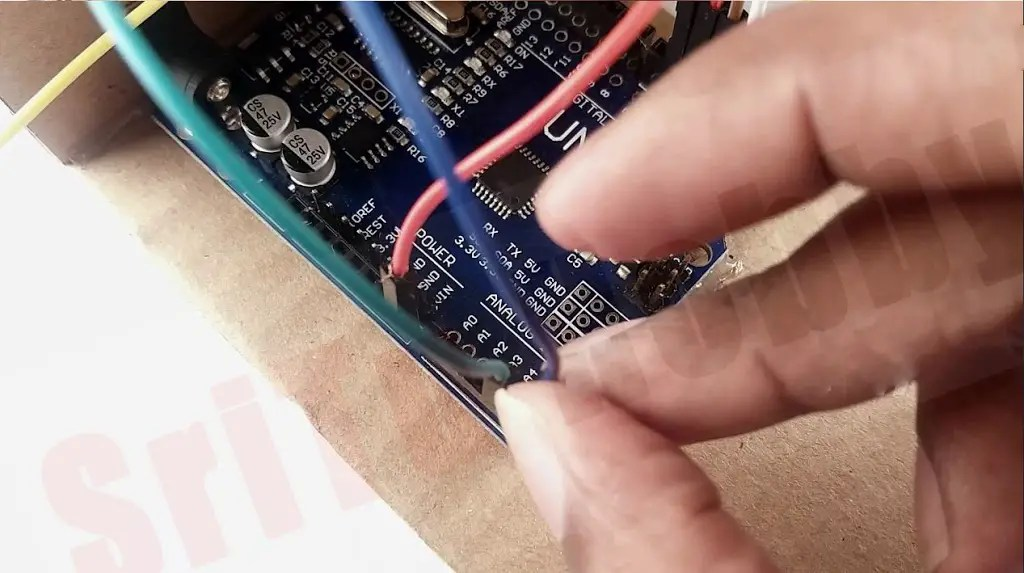### Step 6

Next, cut two 2 * 5-inch pieces and one 1.5* 3-inch piece. After, glue them to the bottom piece of cardboard.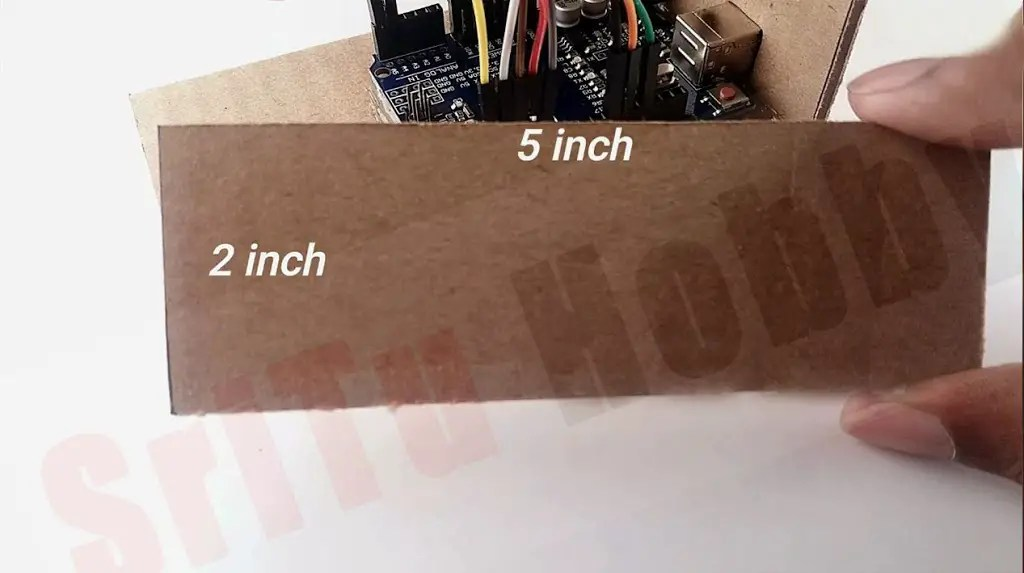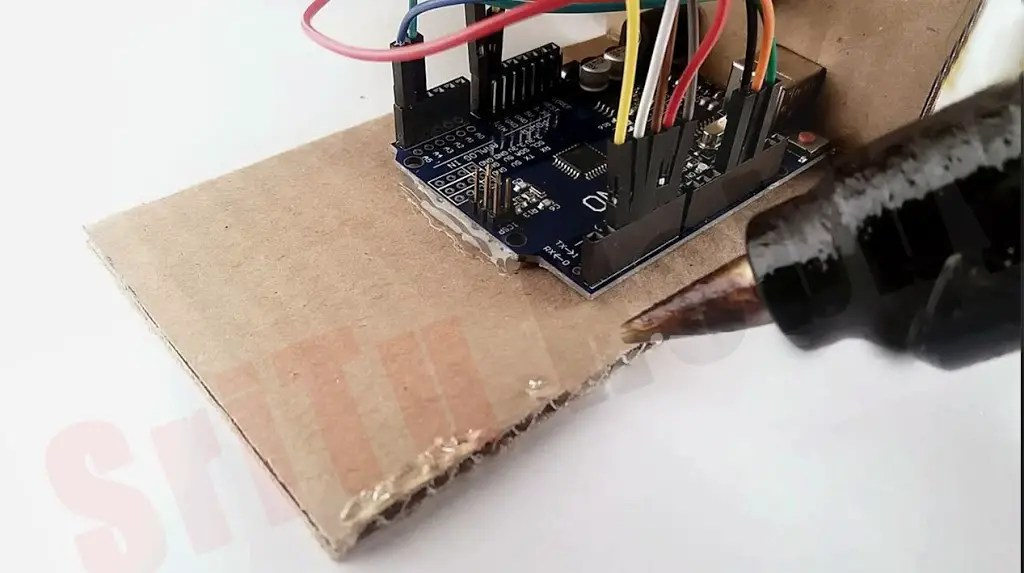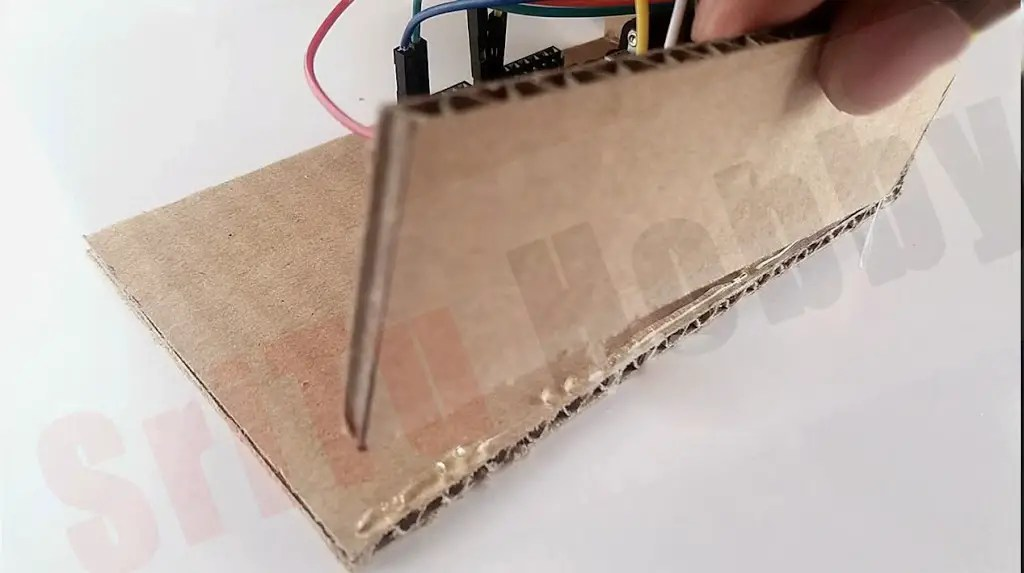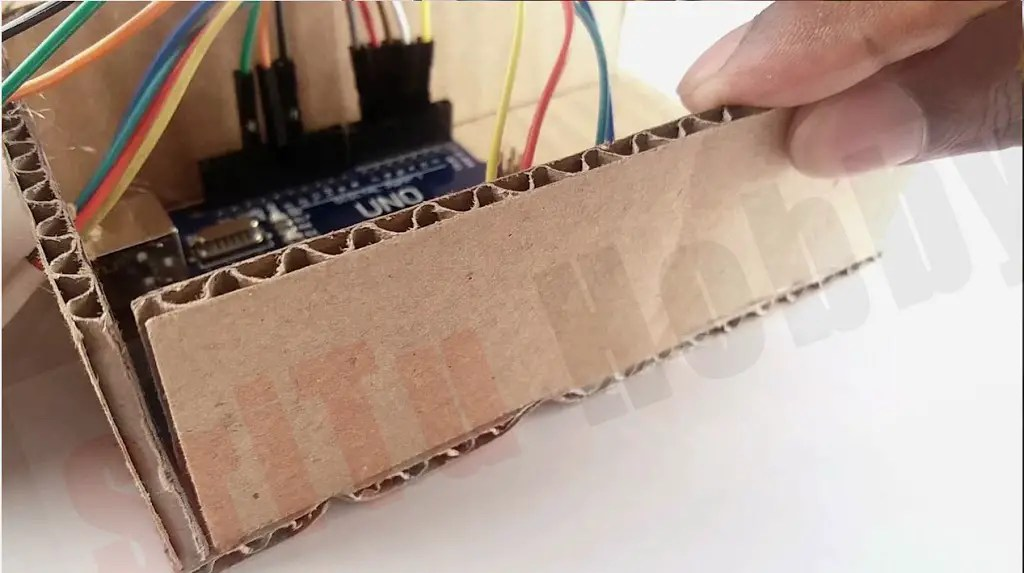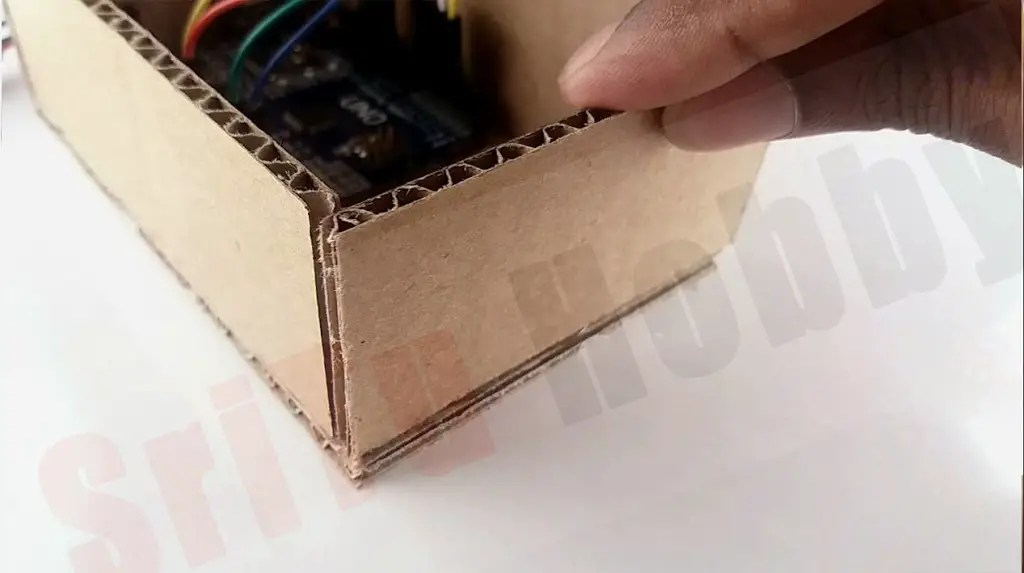### Step 7

Now, glue the LEDs connected cardboard piece to the top.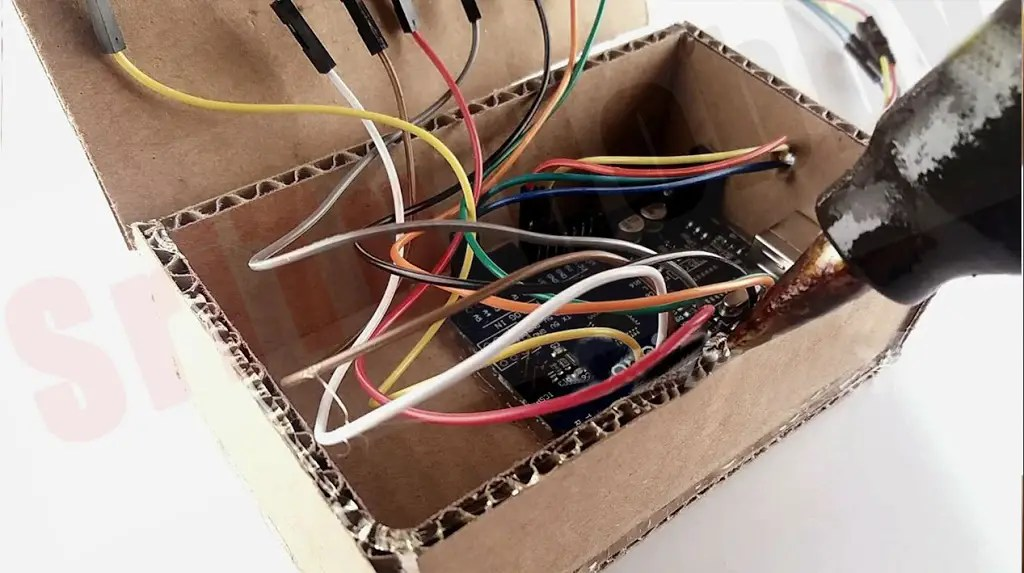### Step 8

OK, now let’s set up the program for this. It is as follows.
``````/*Water level indicator project.
created by the SriTu Tech team.
Read the code below and use it for any of your creations
*/

//define pins and water values
#define Trig A4
#define Echo A5
#define L1 4
#define L2 5
#define L3 6
#define L4 7
#define L5 8
#define L6 10
#define L7 13

int Wlevel;
int range;
int Range;

void setup() {
Serial.begin(9600);//enable serial monitor
pinMode(Trig, OUTPUT);//Ultrasonic Trig pin
pinMode(Echo, INPUT);//Ultrasonic Trig pin
pinMode(2, OUTPUT);//LED pin
pinMode(3, OUTPUT);//LED pin
pinMode(4, OUTPUT);//LED pin
pinMode(5, OUTPUT);//LED pin
pinMode(6, OUTPUT);//LED pin
pinMode(11, OUTPUT);//LED pin
pinMode(12, OUTPUT);//LED pin
}

void loop() {
Range = levelrange();
Serial.println(Range);

//Bulb indicator
if (Range == 1) {
digitalWrite(2, LOW);
analogWrite(3, 50);
digitalWrite(4, LOW);
digitalWrite(5, LOW);
digitalWrite(6, LOW);
digitalWrite(11, LOW);
digitalWrite(12, LOW);
} else if (Range == 2) {
digitalWrite(2, LOW);
digitalWrite(3, HIGH);
digitalWrite(4, HIGH);
digitalWrite(5, LOW);
digitalWrite(6, LOW);
digitalWrite(11, LOW);
digitalWrite(12, LOW);
} else if (Range == 3) {
digitalWrite(2, LOW);
digitalWrite(3, HIGH);
digitalWrite(4, HIGH);
digitalWrite(5, HIGH);
digitalWrite(6, LOW);
digitalWrite(11, LOW);
digitalWrite(12, LOW);
} else if (Range == 4) {
digitalWrite(2, LOW);
digitalWrite(3, HIGH);
digitalWrite(4, HIGH);
digitalWrite(5, HIGH);
digitalWrite(6, HIGH);
digitalWrite(11, LOW);
digitalWrite(12, LOW);
} else if (Range == 5) {
digitalWrite(2, LOW);
digitalWrite(3, HIGH);
digitalWrite(4, HIGH);
digitalWrite(5, HIGH);
digitalWrite(6, HIGH);
digitalWrite(11, HIGH);
digitalWrite(12, LOW);
} else if (Range == 6) {
digitalWrite(2, LOW);
digitalWrite(3, HIGH);
digitalWrite(4, HIGH);
digitalWrite(5, HIGH);
digitalWrite(6, HIGH);
digitalWrite(11, HIGH);
digitalWrite(12, HIGH);
} else if (Range == 000) {
digitalWrite(2, HIGH);
digitalWrite(3, LOW);
digitalWrite(4, LOW);
digitalWrite(5, LOW);
digitalWrite(6, LOW);
digitalWrite(11, LOW);
digitalWrite(12, LOW);
}
}
//check water level range
int levelrange() {
Wlevel = level();
//Serial.println(Wlevel);
if (Wlevel < L7 && Wlevel > L6) {
range = 1;
} else if (Wlevel <= L6 && Wlevel > L5) {
range = 2;
} else if (Wlevel <= L5 && Wlevel > L4) {
range = 3;
} else if (Wlevel <= L4 && Wlevel > L3) {
range = 4;
} else if (Wlevel <= L3 && Wlevel > L2  ) {
range = 5;
} else if (Wlevel <= L2 && Wlevel > L1  ) {
range = 6;
} else if (Wlevel >= L7 ) {
range = 000;
}
return range;
}
int level() {
digitalWrite(Trig, LOW);
delayMicroseconds(4);
digitalWrite(Trig, HIGH);
delayMicroseconds(10);
digitalWrite(Trig, LOW);

long t = pulseIn(Echo, HIGH);//input pulse and save it veriable
long cm = t / 29 / 2; //time convert distance
return cm;
}``````

#### Code explanation.

First, the ultrasonic sensor PIN and water level are defined.
#define Trig A4
#define Echo A5
#define L1 4
#define L2 5
#define L3 6
#define L4 7
#define L5 8
#define L6 10
#define L7 13

Second, these PINs are converted to INPUT and OUTPUT PIN in the void setup.
void setup() {
Serial.begin(9600);//enable serial monitor
pinMode(Trig, OUTPUT);//Ultrasonic Trig pin
pinMode(Echo, INPUT);//Ultrasonic Trig pin
pinMode(2, OUTPUT);//LED pin
pinMode(3, OUTPUT);//LED pin
pinMode(4, OUTPUT);//LED pin
pinMode(5, OUTPUT);//LED pin
pinMode(6, OUTPUT);//LED pin
pinMode(11, OUTPUT);//LED pin
pinMode(12, OUTPUT);//LED pin
}

Thirdly, the LEDs are turned ON and OFF according to the water level in the void loop. The required function for this is prepared below.
void loop() {
Range = levelrange();
Serial.println(Range);

//Bulb indicator
if (Range == 1) {
digitalWrite(2, LOW);
analogWrite(3, 50);
digitalWrite(4, LOW);
digitalWrite(5, LOW);
digitalWrite(6, LOW);
digitalWrite(11, LOW);
digitalWrite(12, LOW);
} else if (Range == 2) {
digitalWrite(2, LOW);
digitalWrite(3, HIGH);
digitalWrite(4, HIGH);
digitalWrite(5, LOW);
digitalWrite(6, LOW);
digitalWrite(11, LOW);
digitalWrite(12, LOW);
} else if (Range == 3) {
digitalWrite(2, LOW);
digitalWrite(3, HIGH);
digitalWrite(4, HIGH);
digitalWrite(5, HIGH);
digitalWrite(6, LOW);
digitalWrite(11, LOW);
digitalWrite(12, LOW);
} else if (Range == 4) {
digitalWrite(2, LOW);
digitalWrite(3, HIGH);
digitalWrite(4, HIGH);
digitalWrite(5, HIGH);
digitalWrite(6, HIGH);
digitalWrite(11, LOW);
digitalWrite(12, LOW);
} else if (Range == 5) {
digitalWrite(2, LOW);
digitalWrite(3, HIGH);
digitalWrite(4, HIGH);
digitalWrite(5, HIGH);
digitalWrite(6, HIGH);
digitalWrite(11, HIGH);
digitalWrite(12, LOW);
} else if (Range == 6) {
digitalWrite(2, LOW);
digitalWrite(3, HIGH);
digitalWrite(4, HIGH);
digitalWrite(5, HIGH);
digitalWrite(6, HIGH);
digitalWrite(11, HIGH);
digitalWrite(12, HIGH);
} else if (Range == 000) {
digitalWrite(2, HIGH);
digitalWrite(3, LOW);
digitalWrite(4, LOW);
digitalWrite(5, LOW);
digitalWrite(6, LOW);
digitalWrite(11, LOW);
digitalWrite(12, LOW);
}
}

This function creates values according to the amount of water.
int levelrange() {
Wlevel = level();
//Serial.println(Wlevel);
if (Wlevel < L7 && Wlevel > L6) {
range = 1;
} else if (Wlevel <= L6 && Wlevel > L5) {
range = 2;
} else if (Wlevel <= L5 && Wlevel > L4) {
range = 3;
} else if (Wlevel <= L4 && Wlevel > L3) {
range = 4;
} else if (Wlevel <= L3 && Wlevel > L2  ) {
range = 5;
} else if (Wlevel <= L2 && Wlevel > L1  ) {
range = 6;
} else if (Wlevel >= L7 ) {
range = 000;
}
return range;
}

This function takes the distance from the ultrasonic sensor.
int level() {
digitalWrite(Trig, LOW);
delayMicroseconds(4);
digitalWrite(Trig, HIGH);
delayMicroseconds(10);
digitalWrite(Trig, LOW);

long t = pulseIn(Echo, HIGH);//input pulse and save it veriable
long cm = t / 29 / 2; //time convert distance
return cm;
}

Step 9
Lastly, select the correct board and port. After, upload this code. For that, connect the Arduino board to the computer.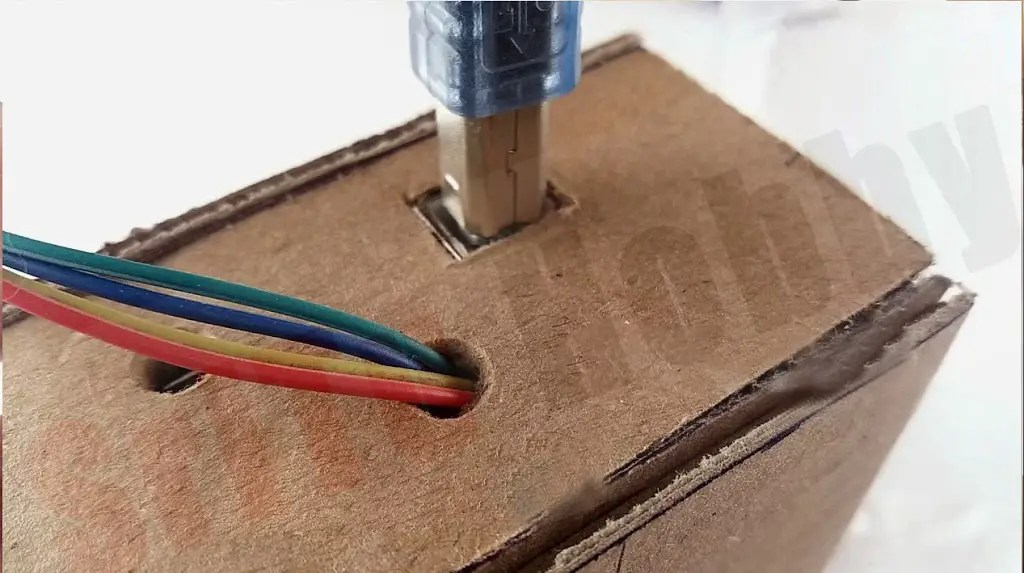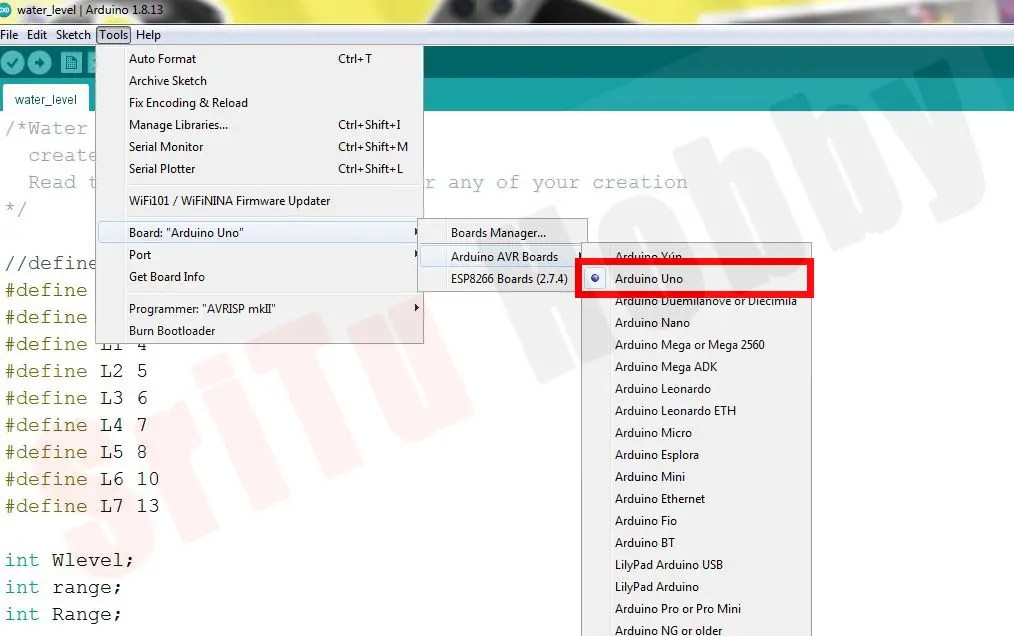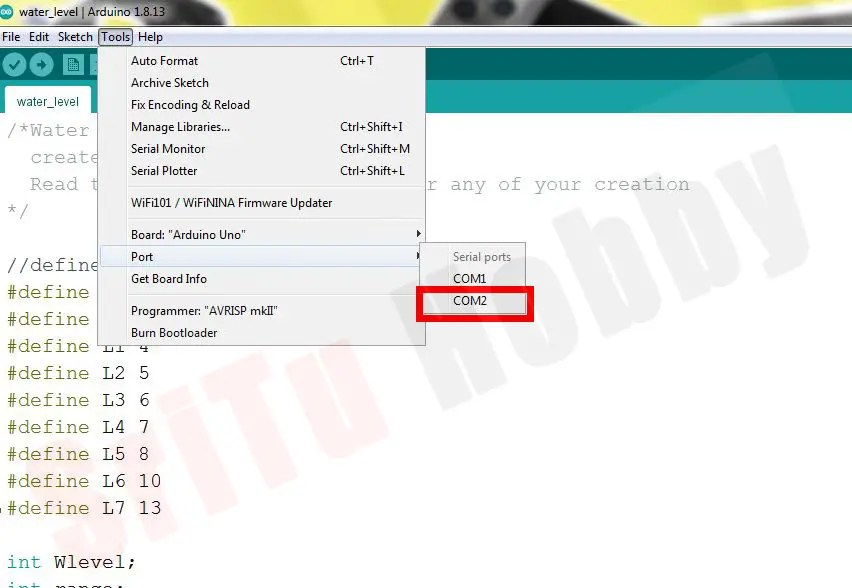### Step 10

OK, now connect the power source to the Arduino board. (You need to remember to give a voltage of 7v to 12v for that). Enjoy this project. The full video guide is given below. We will meet in the next tutorial.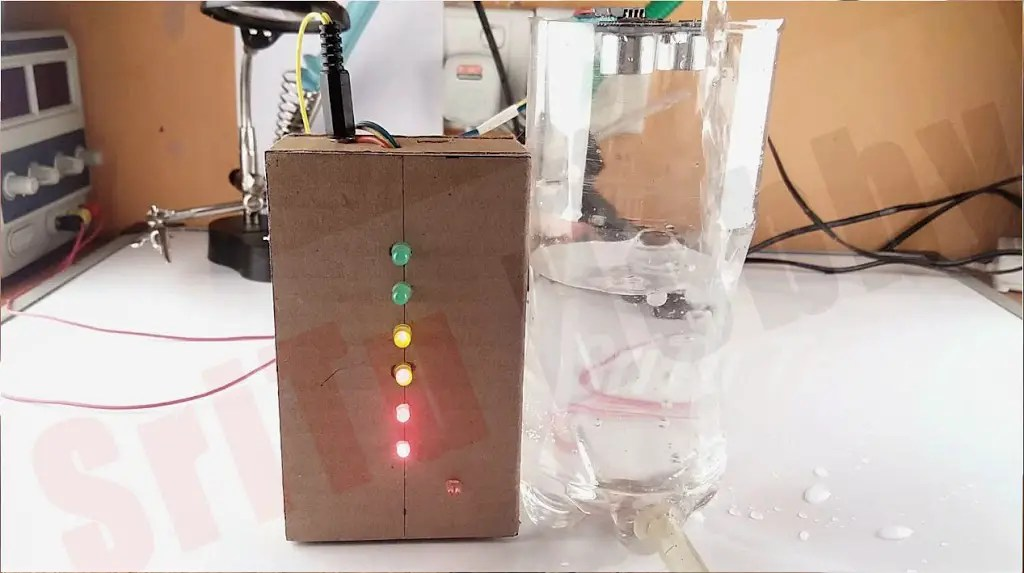How to make a simple water tank level indicator | Step by step instructions